# A Tutorial on Structure Optimized Proximity Scaling (STOPS)

#### 2023-01-16

In this document we introduce the functionality available in stops for fitting multidimensional scaling (MDS; Borg & Groenen 2005) or proximity scaling (PS) models either on their own or by utilizing the STOPS idea. We start with a short introduction to PS and the models that are available. We then explain fitting of these models with the stops package. Next, we introduce the reader to STOPS (Rusch, Mair & Hornik, 2020; Rusch, Mair & Hornik, 2023a), a method to select hyperparameters in flexible mutlidimensional scaling methods based on structures in the configuration. We show how to fit those. We also mention P-COPS as a special case and show the connection to COPS (Rusch, Mair & Hornik, 2021). For illustration throughout this document we use the smacof::kinshipdelta data set (Rosenberg & Kim, 1975) which lists percentages of how often 15 kinship terms were not grouped together by college students.

library(stops)

## Proximity Scaling

For proximity scaling (PS) or multidimensional scaling (MDS) the input is typically an $$N\times N$$ matrix $$\Delta^*=f(\Delta)$$, a matrix of proximities with elements $$\delta^*_{ij}$$, that is a function of a matrix of observed non-negative dissimilarities $$\Delta$$ with elements $$\delta_{ij}$$. $$\Delta^*$$ usually is symmetric (but does not need to be). The main diagonal of $$\Delta$$ is 0. We call a $$f: \delta_{ij} \mapsto \delta^*_{ij}$$ a proximity transformation function. In the MDS literature these $$\delta_{ij}^*$$ are often called dhats or disparities. The problem that proximity scaling solves is to locate an $$N \times M$$ matrix $$X$$ (the configuration) with row vectors $$x_i, i=1,\ldots,N$$ in low-dimensional space $$(\mathbb{R}^M, M \leq N)$$ in such a way that transformations $$g(d_{ij}(X))$$ of the fitted distances $$d_{ij}(X)=d(x_i,x_j)$$—i.e., the distance between different $$x_i, x_j$$—approximate the $$\delta^*_{ij}$$ as closely as possible. We call a $$g: d_{ij}(X) \mapsto d_{ij}^*(X)$$ a distance transformation function. In other words, proximity scaling means finding $$X$$ so that $$d^*_{ij}(X)=g(d_{ij}(X))\approx\delta^*_{ij}=f(\delta_{ij})$$. There may also be weights transformed weight $$w^*_{ij}=h(w_{ij})$$ with $$h: w_{ij} \mapsto w_{ij}^*$$ being a weight transformation function.

This approximation $$D^*(X)$$ to the matrix $$\Delta^*$$ is found by defining a fit criterion (the loss function), $$\sigma_{PS}(X)=L(\Delta^*,D^*(X))$$, that is used to measure how closely $$D^*(X)$$ approximates $$\Delta^*$$.

The loss function used is then minimized to find the vectors $$x_1,\dots,x_N$$, i.e., $\begin{equation} \label{eq:optim} \arg \min_{X}\ \sigma_{PS}(X). \end{equation}$

### Stress Models

The first popular type of PS supported in stops is based on the loss function type stress (Kruskall 1964), employing a quadratic loss function.

A general formulation of a loss function based on a quadratic loss is $\begin{equation} \label{eq:stress} \sigma_{PS}(X)=\sigma_{stress}(X)=\sum^N_{i=1}\sum^N_{j=1} w^*_{ij}\left[d^*_{ij}(X)-\delta^*_{ij}\right]^2=\sum^N_{i=1}\sum^N_{j=1} h(w_{ij})\left[g\left(d_{ij}(X)\right)-f(\delta_{ij})\right]^2 \end{equation}$ Here, the $$w_{ij}$$ are finite weights, with $$w_{ij}=0$$ if the entry is missing and $$w_{ij}=1$$ otherwise. There are a number of optimization techniques one can use to solve this optimization problem.

The distances used is usually some type of Minkowski distance ($$p > 0$$) as the distance fitted to the points in the configuration, $\begin{equation} \label{eq:dist} d_{ij}(X) = ||x_{i}-x_{j}||_p=\left( \sum_{m=1}^M |x_{im}-x_{jm}|^p \right)^{1/p} \ i,j = 1, \dots, N. \end{equation}$ Typically, the norm used is the Euclidean norm, so $$p=2$$. In standard MDS $$g(\cdot)=f(\cdot)=I(\cdot)$$, the identity function.

This formulation enables one to express a large number of PS methods many of which are implemented in stops. In stops we allow to use specific choices for $$f(\cdot)$$, $$g(\cdot)$$ and $$h(\cdot)$$ from the family of power transformations so one can fit the following stress models:

• Explicitly normalized stress: $$w^*_{ij}=(\sum_{ij}\delta^{*2}_{ij})^{-1}$$, $$\delta_{ij}^*=\delta_{ij}$$, $$d^*_{ij}(X)=d_{ij}(X)$$
• Stress-1: $$w^*_{ij}=(\sum_{ij} d^{*2}_{ij}(X))^{-1}$$, $$\delta_{ij}^*=\delta_{ij}$$, $$d_{ij}(X)^*=d_{ij}(X)$$
• Sammon stress (Sammon 1969): $$w^*_{ij}=\delta^{*-1}_{ij}$$ , $$\delta_{ij}^*=\delta_{ij}$$, $$d_{ij}(X)^*=d_{ij}(X)$$
• Elastic scaling stress (McGee 1966): $$w_{ij}=\delta^{*-2}_{ij}$$, $$\delta_{ij}^*=\delta_{ij}$$, $$d_{ij}(X)^*=d_{ij}(X)$$
• S-stress (Takane et al. 1977): $$\delta^*_{ij}=\delta_{ij}^2$$ and $$d^*_{ij}(X)=d^2_{ij}(X)$$, $$w^*_{ij}=1$$
• R-stress (de Leeuw, 2014): $$\delta^*_{ij}=\delta_{ij}$$ and $$d^*_{ij}=d^{2r}_{ij}$$, $$w^*_{ij}=1$$
• Power MDS (Buja et al. 2008, Rusch, Mair & Hornik 2021): $$\delta^*_{ij}=\delta_{ij}^\lambda$$ and $$d^*_{ij}=d^\kappa_{ij}$$, $$w^*_{ij}=1$$
• Power elastic scaling (Rusch, Mair & Hornik 2023): $$w^*_{ij}=\delta^{*-2}_{ij}$$, $$\delta^*_{ij}=\delta_{ij}^\lambda$$ and $$d^*_{ij}=d^\kappa_{ij}$$
• Power Sammon mapping (Rusch, Mair & Hornik 2023): $$w^*_{ij}=\delta^{*-1}_{ij}$$, $$\delta^*_{ij}=\delta_{ij}^\lambda$$ and $$d^*_{ij}=d^\kappa_{ij}$$
• Restricted powerstress (Rusch, Mair & Hornik, 2021): $$\delta^*_{ij}=\delta_{ij}^\lambda$$, $$d^*_{ij}=d^\kappa_{ij}$$ but with the restriction $$\kappa=\lambda$$. The $$w^*_{ij}=w_{ij}^\rho$$ for arbitrary $$w_{ij}$$ (e.g., a function of the $$\delta_{ij}$$)
• Power stress (encompassing all previous models; Buja et al. 2008, Rusch, Mair & Hornik 2021): $$\delta^*_{ij}=\delta_{ij}^\lambda$$, $$d^*_{ij}=d^\kappa_{ij}$$ and $$w^*_{ij}=w_{ij}^\rho$$ for arbitrary $$w_{ij}$$ (e.g., a function of the $$\delta_{ij}$$)
• Approximate power stress (Rusch, Mair & Hornik, 2021): This approximates power stress by $\begin{equation} \label{eq:apstress} \sigma_{PS}(X)=\sigma_{apstress}(X)=\sum_{i<j} \delta_{ij}^\upsilon \left(\delta_{ij}^\tau - d_{ij}(X)\right)^2 \end{equation}$ where the relation to p-stress is so that $$\upsilon=\rho + 2\lambda(1 -1/\kappa)$$ and $$\tau=\lambda/\kappa$$. The approximation of p-stress by ap-stress works well in cases when for the $$x_i,x_j$$ for which $$w_{ij}$$ is large, the error $$\delta_{ij}^\lambda - d_{ij}(X)^\kappa$$ is small, so that $$d_{ij}(X)^\kappa$$ is approximated reasonably well by $$\delta_{ij}^\lambda$$ and, equivalently, $$d_{ij}(X)^\kappa$$ can be approximated well by $$d_{ij}(X) \delta_{ij}^{(\lambda (\kappa-1)/\kappa)}$$.
• Local MDS (LMDS; Chen & Buja, 2009): Let $$N_k$$ define the symmetric set of nearby pairs of points $$(i,j)$$ so that $$(i,j)\in N_k$$ iff $$i$$ is among the $$k-$$nearest neighbours of $$j$$ or the other way round. Then we have a stress model with $\begin{equation} \delta^*_{ij}=\begin{cases} \delta_{ij} &\mbox{ if } (i,j) \in N_k\\ \sqrt{w}\delta_{\infty} &\mbox{ if } (i,j) \in N_k\\ \end{cases} \end{equation}$ and $\begin{equation} d^*_{ij}(X)=\begin{cases} d_{ij}(X) &\mbox{ if } (i,j) \in N_k\\ \sqrt{w}d_{ij}(X) &\mbox{ if } (i,j) \in N_k\\ \end{cases} \end{equation}$ where $$\delta_{\infty} \rightarrow \infty$$ is a large imputed’’ dissimilarity that is constant and $$w$$ a small weight. For simplification, one takes $$w \sim 1/\delta_{\infty}$$ in the standard lMDS objective, so one uses the tuning parameter $$\tau=2w\delta_{\infty}$$ for given $$k$$.

For all of the above models but local MDS one can use the function powerStressMin which uses majorization to find the solution (De Leeuw, 2014) . The function allows to specify a kappa, lambda and nu argument as well as a weightmat (the $$w_{ij}$$). LMDS can be fitted with the lmds function. For approximate power stress there also is a convenience function apStressMin.

Note that the implementation in powerStressMin is more a proof-of-concept than optimal. Majorizing this type of stress is a pretty hard problem and the code we use relies on a while loop in pure R. We plan to speed the loop up with a re-implementation in C in the future.

### Strain Models

The second popular type of PS supported in stops is based on the loss function type . Here the $$\Delta^*$$ are a transformation of the $$\Delta$$, $$\Delta^*= f (\Delta)$$ so that $$f(\cdot)=-(h\circ l)(\cdot)$$ where $$l$$ is any function and $$h(\cdot)$$ is a double centering operation, $$h(\Delta)=\Delta-\Delta_{i.}-\Delta_{.j}+\Delta_{..}$$ where $$\Delta_{i.}, \Delta_{.j}, \Delta_{..}$$ are matrices consisting of the row, column and grand marginal means respectively. These then get approximated by (functions of) the inner product matrices of $$X$$ $\begin{equation} \label{eq:dist2} d_{ij}(X) = \langle x_{i},x_{j} \rangle \end{equation}$ We can thus express classical scaling as a special case of the general PS loss with $$d_{ij}(X)$$ as an inner product, $$g(\cdot) = I(\cdot)$$ and $$f(\cdot)=-(h \circ I)(\cdot)$$.

If we again allow power transformations for $$g(\cdot)$$ and $$f(\cdot)$$ one can fit the following strain models with stops

• Classical scaling (Torgerson, 1958): $$\delta^*_{ij}=-h(\delta_{ij})$$ and $$d^*_{ij}=d_{ij}$$
• Powerstrain (Buja et al. 2008, Rusch, Mair & Hornik 2021): $$\delta^*_{ij}=-h(\delta_{ij}^\lambda)$$, $$d^*_{ij}=d_{ij}$$ and $$w_{ij}=w_{ij}^\rho$$ for arbitrary $$w_{ij}$$

In stops we have a wrapper to cmdscale (overloading the base function) which extend functionality by offering an object that matches smacofP objects with corresponding methods.

### Energy Models

The third type of PS supported in stops is based on the energy type losses of pairwise attraction and repulsion between objects (Chen & Buja, 2014, Rusch, Mair, Hornik 2023a). It is related to graph drawing: if vertices are equated with objects, the edge weights are the $$\delta^*_{ij}$$, the node positions in the layout is the $$X$$ and the distance between nodes in the layout are $$d_{ij}(X)$$.

One can express badness-of-fit as comprising a repulsion part $$\propto -\delta^*_{ij}d^*_{ij}(X)$$ and an attraction part $$\propto d^{*\mu}_{ij}(X)$$ (Chen & Buja, 2014). This is
$\begin{equation} \label{eq:energy} \sigma_{PS}(X)=\sigma_{EM}(X) = \sum_{i<j} w^*_{ij} \left(a d^{*\mu}_{ij}(X) - b \delta^*_{ij}d^*_{ij}(X) \right) \end{equation}$ with $$a,b$$ being constants and $$d^*_{ij}(X)=g(d_{ij}(X))$$ and $$\delta^*_{ij}=f(d_{ij}(X))$$. For $$\mu=2, a=1$$ and $$b=2$$ this is a stress model where terms depending solely on $$\delta^*_{ij}$$ are disregarded for finding $$X$$.

Specific instances of energy models that can be fitted in stops are:

• Box-Cox MDS (Chen & Buja, 2014). This is a three-parameter family $$\theta=(\mu^{(BC)},\lambda^{(BC)},\rho^{(BC)})^\top$$: $\begin{equation} \label{eq:bcstress} \sigma_{PS}(X)=\sigma_{BCstress}(X) = \sum_{i<j} \delta_{ij}^{\rho} \left( BC_{\mu+\lambda}(d_{ij}(X)) - \delta_{ij}^{\lambda} BC_{\mu}(d_{ij}(X)) \right) \end{equation}$ with $$\mu, \rho\in \mathbb{R}$$ and $$\lambda \in \mathbb{R}_+$$. Here $$BC_\alpha$$ is the one-parameter Box-Cox transformation with parameter $$\alpha$$, $\begin{equation} \label{eq:bc} BC_{\alpha}(d)=\begin{cases} \frac{d^{\alpha}-1}{\alpha} \mbox{ if } \alpha \neq 0\\ \log(d) \mbox{ if } \alpha = 0\\ \end{cases} \end{equation}$ For $$w_{ij}=\delta_{ij}, \mu=\lambda=1$$ this is very similar to the stress-equivalent energy MDS model. Note that here the distance transformation functions in the attraction and repulsion parts need not be equal. $$\sigma_{\text{BC}}(X)$$ shares close similarities with stress with power transformations (power stress): They will often lead to a similar configurations for the same parameters (even equivalent if the exponents for repulsion and attraction parts are the same).

### Usage of flexible MDS models

The objects returned from powerStressMin, lmds, bcStressMin and apStressMin inherit from class smacofP which extends the smacof classes (De Leeuw & Mair, 2009) to allow for the transformations. Apart from that all the objects are made so that they are compatible to methods from smacof and also inherit from smacof. Accordingly, the following S3 methods are available:

Method Description
print Prints the object
summary A summary of the object
plot 2D Plots of the object
plot3d Dynamic 3D configuration plot
plot3dstatic Static 3D configuration plot
residuals Residuals
coef Model Coefficients

Let us illustrate the usage.

First we setup our dissimilarity matrix $$[\delta_{ij}]$$ and call it dis.

dis<-as.matrix(smacof::kinshipdelta)

We now fit a series of MDS models available in stops.

• A standard MDS (stress)
res1<-powerStressMin(dis,kappa=1,lambda=1)
res1
##
## Call:
## powerStressMin(delta = dis, kappa = 1, lambda = 1)
##
## Model: Power Stress SMACOF
## Number of objects: 15
## Stress-1 value: 0.264
## Number of iterations: 5352
• A sammon mapping
res2<-powerStressMin(dis,kappa=1,lambda=1,nu=-1,weightmat=dis)
res2
##
## Call:
## powerStressMin(delta = dis, kappa = 1, lambda = 1, nu = -1, weightmat = dis)
##
## Model: Power Stress SMACOF
## Number of objects: 15
## Stress-1 value: 0.289
## Number of iterations: 81666

Alternatively, one can use the faster sammon function from MASS (Venables & Ripley, 2002) for which we provide a wrapper that adds class attributes and methods (and overloads the function).

res2a<-sammon(dis)
## Initial stress        : 0.17053
## stress after   3 iters: 0.10649
res2a
##
## Call: sammon(d = dis)
##
## Model: Sammon Scaling
## Number of objects: 15
## Stress: 0.1064925
• An elastic scaling
res3<-powerStressMin(dis,kappa=1,lambda=1,nu=-2,weightmat=dis)
res3
##
## Call:
## powerStressMin(delta = dis, kappa = 1, lambda = 1, nu = -2, weightmat = dis)
##
## Model: Power Stress SMACOF
## Number of objects: 15
## Stress-1 value: 0.305
## Number of iterations: 1e+05
• A sstress model
res4<-powerStressMin(dis,kappa=2,lambda=2)
res4
##
## Call:
## powerStressMin(delta = dis, kappa = 2, lambda = 2)
##
## Model: Power Stress SMACOF
## Number of objects: 15
## Stress-1 value: 0.346
## Number of iterations: 47130
• An rstress model (with $$r=1$$ as $$r=\kappa/2$$)
res5<-powerStressMin(dis,kappa=2,lambda=1)
res5
##
## Call:
## powerStressMin(delta = dis, kappa = 2, lambda = 1)
##
## Model: Power Stress SMACOF
## Number of objects: 15
## Stress-1 value: 0.404
## Number of iterations: 21201
• A powermds model (i.e., $$\rho=1$$)
res6<-powerStressMin(dis,kappa=2,lambda=1.5)
res6
##
## Call:
## powerStressMin(delta = dis, kappa = 2, lambda = 1.5)
##
## Model: Power Stress SMACOF
## Number of objects: 15
## Stress-1 value: 0.367
## Number of iterations: 50564
• A powersammon model
res7<-powerStressMin(dis,kappa=2,lambda=1.5,nu=-1,weightmat=dis)
res7
##
## Call:
## powerStressMin(delta = dis, kappa = 2, lambda = 1.5, nu = -1,
##     weightmat = dis)
##
## Model: Power Stress SMACOF
## Number of objects: 15
## Stress-1 value: 0.436
## Number of iterations: 1e+05
• A powerelastic scaling
res8<-powerStressMin(dis,kappa=2,lambda=1.5,nu=-2,weightmat=dis)
res8
##
## Call:
## powerStressMin(delta = dis, kappa = 2, lambda = 1.5, nu = -2,
##     weightmat = dis)
##
## Model: Power Stress SMACOF
## Number of objects: 15
## Stress-1 value: 0.519
## Number of iterations: 1e+05
• A powerstress model
res9<-powerStressMin(dis,kappa=2,lambda=1.5,nu=-1.5,weightmat=2*1-diag(nrow(dis)))
res9
##
## Call:
## powerStressMin(delta = dis, kappa = 2, lambda = 1.5, nu = -1.5,
##     weightmat = 2 * 1 - diag(nrow(dis)))
##
## Model: Power Stress SMACOF
## Number of objects: 15
## Stress-1 value: 0.367
## Number of iterations: 57237
summary(res9)
##
## Configurations:
##                    D1      D2
## Aunt          -0.1225  0.2498
## Brother        0.1964 -0.1400
## Cousin         0.0525  0.3099
## Daughter      -0.2050 -0.1256
## Father         0.1639 -0.1822
## Granddaughter -0.2358 -0.0531
## Grandfather    0.2146 -0.1336
## Grandmother   -0.2360 -0.0868
## Grandson       0.2147 -0.1079
## Mother        -0.2098 -0.1363
## Nephew         0.1707  0.2110
## Niece         -0.1231  0.2442
## Sister        -0.2219 -0.0967
## Son            0.1702 -0.1707
## Uncle          0.1710  0.2181
##
##
## Stress per point:
##                  SPP SPP(%)
## Niece         0.0008 4.6861
## Nephew        0.0008 4.7097
## Aunt          0.0008 4.9367
## Uncle         0.0008 5.0269
## Daughter      0.0010 6.0875
## Son           0.0010 6.2785
## Father        0.0011 6.4569
## Mother        0.0011 6.6921
## Cousin        0.0011 6.8096
## Sister        0.0012 7.2765
## Brother       0.0012 7.2882
## Grandson      0.0013 7.9701
## Granddaughter 0.0013 8.0950
## Grandmother   0.0015 8.8103
## Grandfather   0.0015 8.8761
• An lmds model
res10<-lmds(dis,tau=0.5,k=3)
res10
##
## Call:
## lmds(delta = dis, k = 3, tau = 0.5)
##
## Model: Local MDS
## Number of objects: 15
## Stress-1 value: 0.302
## Number of iterations: 80
• An apstress model (approximate power stress)
res11<-apStressMin(dis,tau=0.5,ups=2)
res11
##
## Call:
## apStressMin(delta = dis, tau = 0.5, ups = 2)
##
## Model: Approximate Power Stress SMACOF
## Number of objects: 15
## Stress-1 value: 0.217
## Number of iterations: 16

We can visualize the obtained configurations with plot. For smacofP objects we have the following plots.

plot(res9)
plot(res9,"transplot")
plot(res9,"Shepard")
plot(res9,"resplot")
plot(res9,"bubbleplot")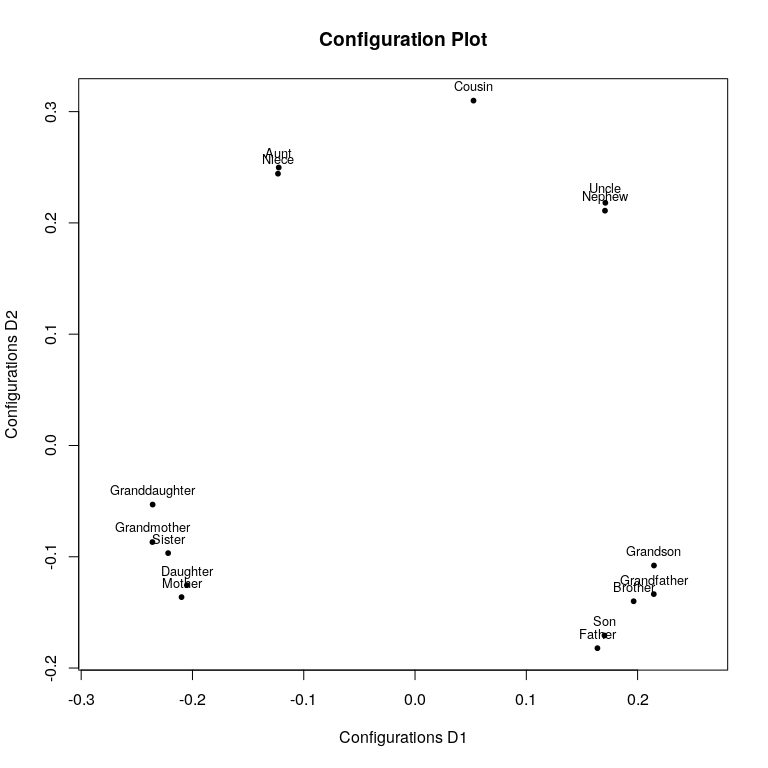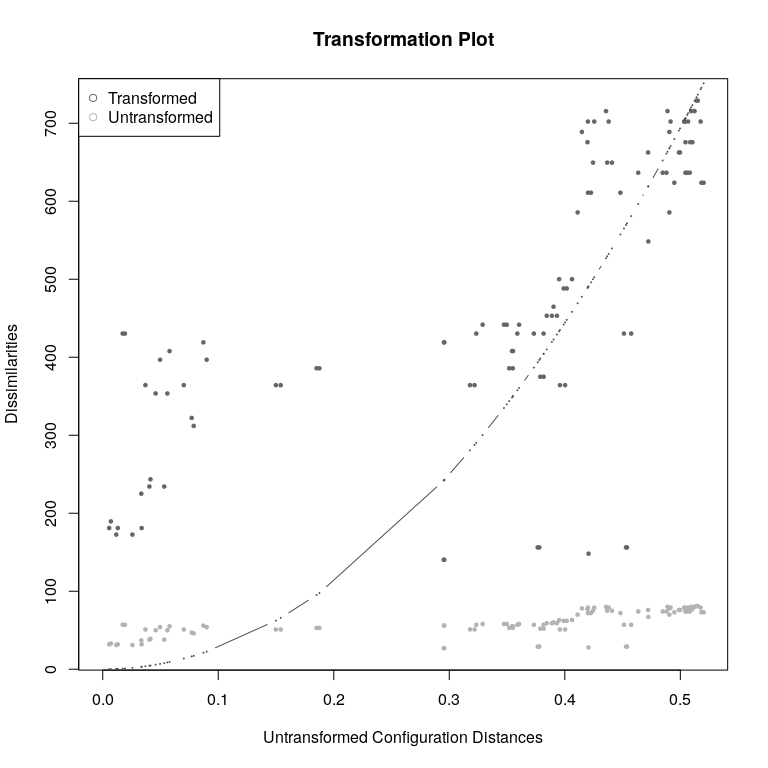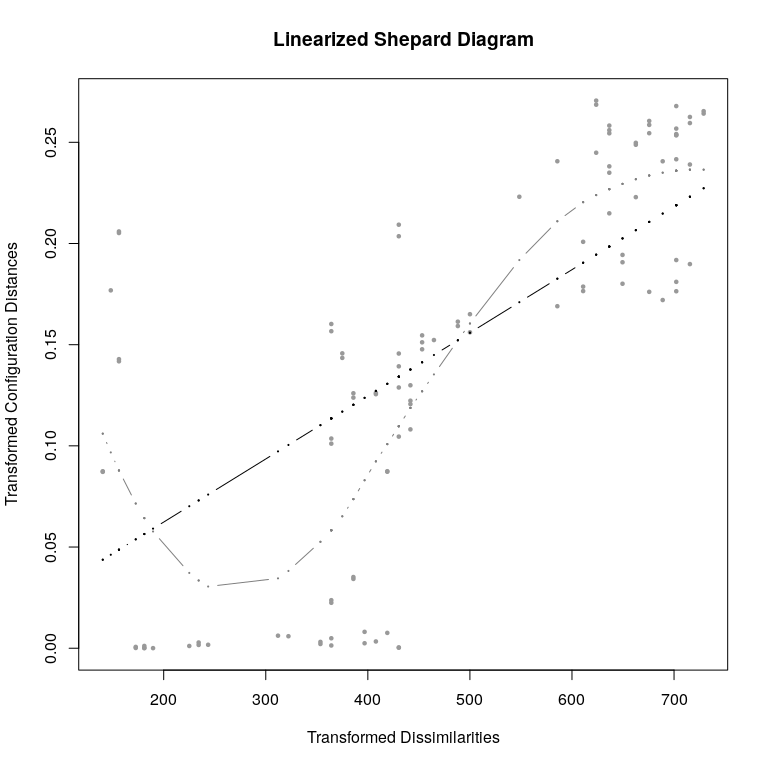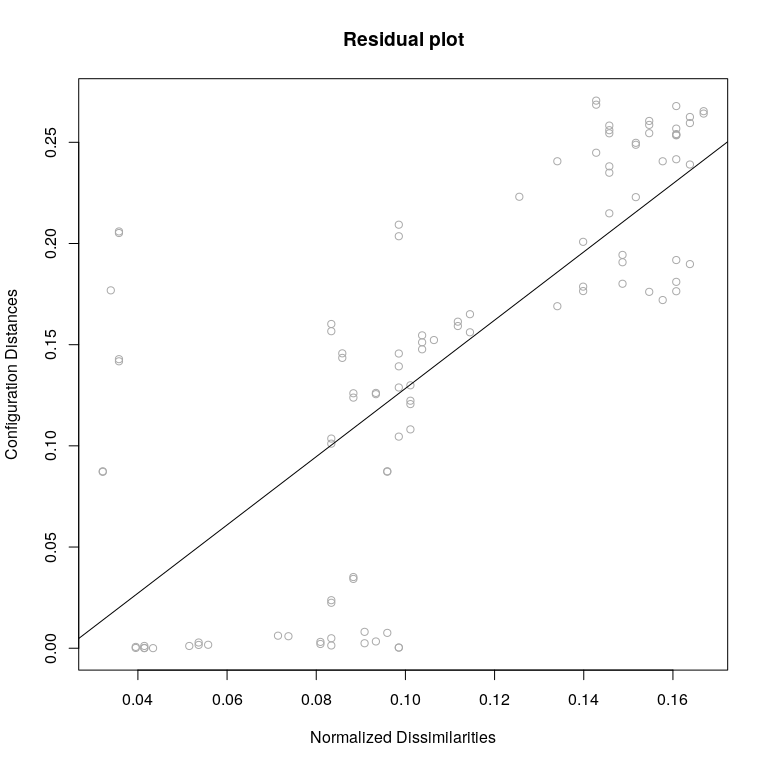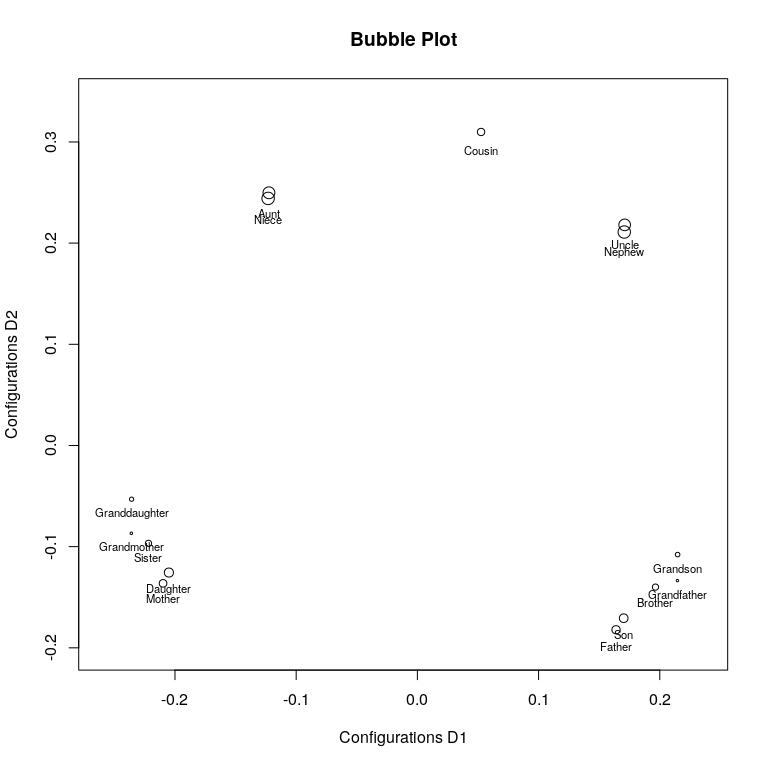• powerstrain model is rather easy to fit with simply subjecting the dissimilarity matrix to some power. Here we use $$\lambda=3$$.
resc<-cmdscale(kinshipdelta^3)
resc
##
## Call: cmdscale(d = kinshipdelta^3)
##
## Model: Torgerson-Gower Scaling
## Number of objects: 15
## GOF: 0.4257747 0.6281985
summary(resc)
##
## Configurations:
##                       D1         D2
## Aunt           178193.10  204986.70
## Brother       -174369.39  -94357.29
## Cousin         -48355.46  265057.17
## Daughter       169149.17 -109936.27
## Father        -145389.13 -168604.23
## Granddaughter  187039.12  -44850.80
## Grandfather   -180116.08 -103668.07
## Grandmother    199145.19  -83038.71
## Grandson      -169767.75  -72358.82
## Mother         185797.71 -138677.31
## Nephew        -195963.98  173123.81
## Niece          168278.13  208870.31
## Sister         182224.16  -70764.01
## Son           -149876.11 -135883.18
## Uncle         -205988.70  170100.68
summary(resc)
plot(resc)
• A BC MDS with bcStressMin
resbc<-bcStressMin(dis,mu=3,lambda=2,nu=2)
resbc
##
## Call:
## bcStressMin(delta = dis, mu = 3, lambda = 2, nu = 2)
##
## Model: Box-Cox Stress MDS
## Number of objects: 15
## Stress-1 value: 0.251
## Number of iterations: 67
plot(resbc)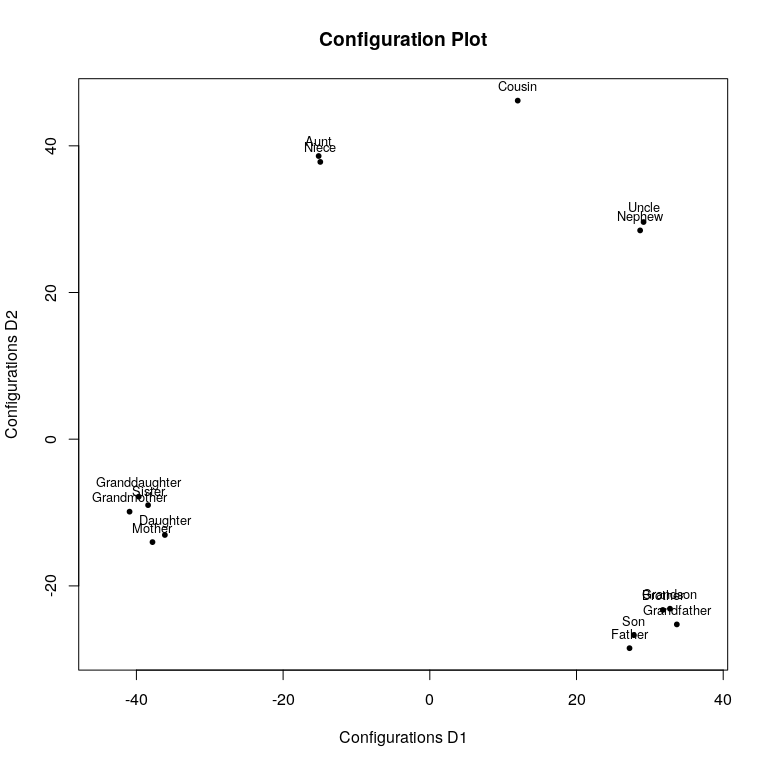## Selecting Hyperparameters by Structure Considerations: STOPS

We saw that these flexible models from above ( power stress, power strain, approximate power stress, local MDS, Box-Cox MDS and their variants) all have hyperparameters $$\theta$$; for example, the hyperparameter vector for power stress is $$\theta=(\kappa,\lambda,\rho)$$. That begs the question which hyperparameters should be chosenas the chosen values have a huge impact on how the final configuration looks like (clearly a $$kappa=2$$ will lead to a different configuration than $$\kappa=0.5$$). So far, we have simply assumed the analyst knows the “right” $$\theta$$; indeed often (and as we did so far) they are simply chosen ad hoc.

The main contribution of the stops package is not solely in fitting the flexible MDS models for given $$\theta$$. The idea behind STOPS is to also allow to automatically select good hyperparameters $$\theta^*$$ to achieve a “structured” MDS configuration. This can be useful in a variety of contexts: to explore or generate structures, to restrict the target space, to avoid artifacts, to preserve certain types of structures and so forth.

For this, the flexible MDS loss functions as described above are augmented to include penalties for the type of structures one is aiming for. This combination of an MDS loss with a structuredness penalty is what we call “structure optimized loss” (stoploss) and the resulting method is coined “Structured Optimized Proximity Scaling” (or STOPS, Rusch, Mair & Hornik, 2023a). STOPS is related to “Cluster Optimized Proximity Scaling” (COPS; Rusch, Mair & Hornik, 2021) which allows to select optimal parameters so that the clusteredness appearance of the configuation is improved (see below).

### Model

Following Rusch, Mair & Hornik (2023a) the general idea is that from given observations $$\Delta$$ we look for a configuration $$X$$. The $$X$$ has properties with regards to its structural appearance, which we call c-structuredness for configuration-structuredness. There are different types of c-structuredness people might be interested in (say, how clustered the result is, or that dimensions are orthogonal or if there is some submanifold that the data live on). We developed indices for these types of c-structuredness that capture that essence in the configuration (see Rusch, Mair & Hornik 2023b, or Rusch, Mair & Hornik 2020 for a large list of structures and indices).

We have as part of a STOPS model a proximity scaling loss function $$\sigma_{PS}(\cdot)$$ and some transformation $$f_{ij}(\delta_{ij}|\theta_f)$$ and $$g_{ij}(d_{ij}|\theta_g)$$ and possibly $$h_{ij}(w_{ij}|\theta_h)$$. These functions are parametrized and all parameters are collected in the vector $$\theta=(\theta_f,\theta_g,\theta_h)$$. $$\theta$$ is finite, e.g., a transformation applied to all observations like a power transformation. For example for power stress $$\theta=(\kappa,\lambda,\rho)$$ and for Box Cox MDS it is $$\theta=(\mu,\lambda,\rho)$$. These transformations achieve a sort of push towards more structure, so different values for $$\theta$$ will in general lead to different c-structuredness.

Let us assume we are interested in $$L$$ different structural qualities of $$X$$ and that we have $$L$$ corresponding univariate c-structuredness indices $$I_l(X\vert \gamma)$$ for the $$l=1,\dots, L$$ different structures, capturing the essence of the structural appearance of the configuration with respect to the $$l$$-th structure. For example, we might be interested in both the structural appearance of how clustered the configuration is (structure 1) and how strongly linearly related the column vectors of the configuration are (structure 2). We then measure the c-structuredness of $$X$$ for the two structures with an index for clusteredness and one for linear dependence respectively. The $$\gamma$$ are optional metaparameters for the indices, which we assume are given and fixed; they control how c-structuredness is measured. We further assume broadly that the transformations $$f(\Delta\vert\theta_f)$$ and/or $$g(D(X)\vert \theta_g)$$ and/or $$h(W\vert \theta_h)$$ produce different c-structuredness in $$X$$ for different values of $$\theta$$.

In a nutshell our proposal is to select optimal hyperparameters $$\theta^\ast$$ for the scaling procedure by assessing the c-structuredness of an optimal configuration $$X^\ast$$ found from a PS method for given $$\theta$$ usually in combination with its badness-of-fit value. We aim at finding a $$\theta^\ast$$ that, when used as transformation parameters in the PS method, will give a configuration that has high (or low) values of the c-structuredness indices. We view this as a multi-objective optimization problem, where we want to maximize/minimize different criteria (either badness-of-fit, or c-structuredness, or both) over $$\theta$$. C-structuredness may this way be induced at a possible expense of fit but we control the expense amount.

To formalize this we explicitly write the building blocks of the objective function used for hyperparameter tuning via STOPS as a function of $$\theta$$: Let us denote by $$X^\ast(\theta)$$ the optimal solution from minimizing a badness-of-fit $$\sigma_{PS}(X \vert \theta)$$ for a fixed $$\theta$$, so $$X^\ast(\theta):= \arg\min_{X} \sigma_{PS}(X\vert \theta)$$. Further we also have the $$L$$ different univariate indices with possible metaparameters $$\gamma$$, $$I_l(X^\ast(\theta)\vert \gamma)$$ to be optimized for.

Specific variants of STOPS can be instantiated by defining objective functions $$\text{Stoploss}(\theta\vert v_0, \dots, v_L, \gamma)$$, comprising either badness-of-fit or c-structuredness indices or both in a scalarized combination. Two variants of objective functions are of the following form:

• Additive STOPS (aSTOPS) $\begin{equation} \label{eq:astops} \text{Stoploss}_{aSTOPS}(\theta\vert v_0, \dots, v_L, \gamma) = v_0 \cdot \sigma_{PS}(X^\ast(\theta)\vert\theta) + \sum^L_{l=1} v_l I_l(X^\ast(\theta)\vert\gamma) \end{equation}$

• Multiplicative STOPS (mSTOPS) $\begin{equation} \label{eq:mstops} \text{Stoploss}_{mSTOPS}(\theta\vert v_0, \dots, v_L, \gamma) = \sigma_{PS}(X^\ast(\theta)\vert\theta)^{v_0} \cdot \prod^L_{l=1} I_l(X^\ast(\theta)\vert\gamma)^{v_l} \end{equation}$

with $$v_0 \in \mathbb{R}_{\geq 0}$$ and $$v_1,\dots,v_L \in \mathbb{R}$$ being the scalarization weights that determine how the individual parts (MDS loss and c-structuredness indices) are aggregated. Note that in this formulation the aggregation is a sum/product, so the weights must be negative if a higher index stands for more structure and we want more of that structure. Alternatively, the stressweight can be negative and the strucweight positive.

Numerically, the badness-of-fit function value $$\sigma_{PS}(X^\ast(\theta)\vert \theta)$$ needs to be normalized to be scale-free and commensurable for comparability of different values of $$\theta$$. The objective function for aSTOPS is fully compensatory, whereas for mSTOPS it ensures that a normalized badness-of-fit of $$0$$ will always lead to the minimal $$\text{Stoploss}(\theta\vert v_0, \dots, v_L, \gamma)$$ for a positive value of $$I_l(\cdot)$$. For notational convenience, we will refer to the objective functions for STOPS variants by $$\text{Stoploss}(\theta)$$ from now on.

The job is then to find $\begin{equation} \arg\min_{\theta}\ \text{Stoploss}(\theta) \end{equation}$

Minimizing stoploss can be quite difficult. In stops we use a nested algorithm combining optimization that internally first solves for $$X$$ given $$\theta$$, $$\arg\min_X \sigma_{PS}\left(X|\theta\right)$$ in an inner loop, and then optimize over $$\theta$$ with a metaheuristic in an outer loop. The number of iterations of the outer loop can be controlled with itmax, the number of iterations of the inner loop with itmaxps.

#### Optimization

Implemented metaheuristics are simulated annealing (optimmethod="SANN"), particle swarm optimization (optimmethod="pso"), DIRECT (optimmethod="DIRECT"), stochastic global optimization (optimmethod="stogo"), COBYLA (optimmethod="cobyla"), Controlled Random Search 2 with local mutation (optimmethod="crs2lm"), Improved Stochastic Ranking Evolution Strategy (optimmethod="isres"), Multi-Level Single-Linkage (optimmethod="mlsl"), Nelder-Mead (optimmethod="neldermead"), Subplex (optimmethod="sbplx"), Hooke-Jeeves Pattern Search (optimmethod="hjk"), CMA-ES (optimmethod="cmaes"), Bayesian optimization with Gaussian Process priors and Kriging (optimmethod="Kriging"), Bayesian optimization with treed Gaussian processes with jump to linear models (optimmethod="tgp") and Adaptive Luus-Jaakola Search (optimmethod="ALJ"). Defaults is “ALJ”.

So there are a lot of solvers to choose from. In our experience tgp, ALJ, Kriging and pso usually work reasonably well for relatively low values of itmax, the iterations of the outer loop (up to 20). If the data are small, then ALJ and pso (with s=5 particles) and with relatively high itmax (>50) is typically good. If the data are larger (so that solving the PS problem is becoming very costly) we want to minimize the number of outer iterations and then Bayesian optimization with tgp or Kriging is typically good for itmax of about $$10$$. The return of tgp is diminishing for higher itmax, so if a higher number of itmax can be afforded pso is often better and more efficient.

#### C-Structuredness

Currently the following c-structuredness types are supported:

• c-clusteredness (cclusteredness): A clustered appearance of the configuration ($$I_k$$ is the normed OPTICS cordillera (COPS; Rusch et al. 2015a); 0 means no c-clusteredness, 1 means perfect c-clusteredness)
• c-linearity (clinearity): Projections lie close to a linear subspace of the configuration ($$I_k$$ is maximal multiple correlation; 0 means orthogonal, 1 means perfectly linear)
• c-manifoldness (cmanifoldness): Projections lie on a sub manifold of the configuration ($$I_k$$ is maximal correlation (Sarmanov, 1958); only available for two dimensions; 1 means perfectly smooth function)
• c-dependence (cdependence): Random vectors of projections onto the axes are stochastically dependent ($$I_k$$ is distance correlation (Szekely et al., 2007); only available for two dimensions; 0 means they are stochastically independent)
• c-association (cassociation): Pairwise nonlinear association between dimensions ($$I_k$$ is the pairwise maximal maximum information coefficient (Reshef et al. 2011), 1 means perfect functional association)
• c-nonmonotonicity (cnonmonotonicity): Deviation from monotonicity ($$I_k$$ is the pairwise maximal maximum assymmetry score (Reshef et al. 2011), the higher the less monotone)
• c-functionality (cfunctionality): Pairwise functional, smooth, noise-free relationship between dimensions ($$I_k$$ is the mean pairwise maximum edge value (Reshef et al. 2011), 1 means perfect functional association)
• c-complexity (ccomplexity): Measures the degree of complexity of the functional relationship between any two dimensions ($$I_k$$ is the pairwise maximal minimum cell number (Reshef et al. 2011), the higher the more complex)
• c-faithfulness (cfaithfulness): How accurate is the neighbourhood of $$\Delta$$ preserved in $$D$$ ($$I_k$$ is the adjusted Md index of Chen & Buja, 2013; note that this index deviates from the others by being a function of both $$X^*$$ and $$\Delta^*$$ rather than $$X^*$$ alone)
• c-regularity (cregularity): How regular are the objects arranged.
• c-hierarchy (chierachy): Captures how well a partition/ultrametric (obtained by hclust) explains the configuration distances.
• c-convexity (cconvexity): Measures how convex the object arrangement is.
• c-striatedness (cstriatedness): Measures how striated the object arrangement is.
• c-outlying (coutlying): Measures if there are many outlying points.
• c-skinniness (cskinniness): Measures how skinny the object arrangement is.
• c-sparsity (csparsity): Measures how sparse the object arrangement is.
• c-stringiness (cstringiness): Measures how stringy the object arrangement is.
• c-clumpiness (cclumpiness): Measures how clumpy the object arrangement is.
• c-inequality (cinequlaity): Measures how unequal the object arrangement is (as it is the Pearson coefficient of variation)

See Rusch, Mair, Hornik (2020) or Rusch, Mair, Hornik (2023b) for a precise definition of each c-structuredness index.

One can also call each index with the function c_foo where foo stands for the structure, so e.g. c_stringiness(X) will give the value of c-stringiness for object X. Note that the c-structuredness functions may have additional metaparameters. For example:

X<-res9$conf c_stringiness(X) ##  1 c_clumpiness(X) ##  0.5944404 c_association(X,alpha=0.7,C=10) ##  0.4370104 c_clusteredness(X,minpts=3) ##  0.5546788 If we have a single $$I(X)=OC(X)$$, the OPTICS cordillera (Rusch, Hornik & Mair 2018), and the transformations applied are power transformations and the weights for the $$I(X)$$ is negative we essentially have P-COPS (see below). #### MDS models for STOPS For the MDS loss (argument loss in functions stops), the functions currently support all losses derived from powerstress, powerstrain, lmds and bcStress. We offer dedicated functions that either use workhorses that are more optimized for the problem at hand and/or restrict the parameter space for the distance/proximity transformations and thus can be faster. They are: • stress, smacofSym: Kruskal’s stress; Workhorse: smacofSym, Optimization over $$\theta=\lambda$$ • smacofSphere: Kruskal’s stress for projection onto a sphere; Workhorse smacofSphere, Optimization over $$\theta=\lambda$$ • strain, powerstrain: Classical scaling; Workhorse: cmdscale, Optimization over $$\theta=\lambda$$ • sammon, sammon2: Sammon scaling; Workhorse: sammon or smacofSym, Optimization over $$\theta=\lambda$$ • elastic: Elastic scaling; Workhorse: smacofSym, Optimization over $$\theta=\lambda$$ • sstress: S-stress; Workhorse: powerStressMin, Optimization over $$\theta=\lambda$$ • rstress: S-stress; Workhorse: powerStressMin, Optimization over $$\theta=\kappa$$ • powermds: MDS with powers; Workhorse: powerStressMin, Optimization over $$\theta=(\kappa,\lambda)$$ • powersammon: Sammon scaling with powers; Workhorse: powerStressMin, Optimization over $$\theta=(\kappa,\lambda)$$ • powerelastic: Elastic scaling with powers; Workhorse: powerStressMin, Optimization over $$\theta=(\kappa,\lambda)$$ • powerstress: Power stress model; Workhorse: powerStressMin, Optimization over $$\theta=(\kappa,\lambda,\rho)$$ • rpowerstress: restricted power stress model; Workhorse: powerStressMin, Optimization over $$\theta=(\kappa,\lambda,\rho)$$ • apstress: Approximate power stress model; Workhorse: powerStressMin, Optimization over $$\theta=(\tau,\upsilon)$$ • lmds: LMDS; Workhorse: lmds, Optimization over $$\theta=(\tau, k)$$. • bcstress: Box-Cox MDS; Workhorse: bcStressMin, Optimization over $$\theta=(\mu,\lambda,\rho)$$. #### Usage The syntax for fitting a stops model is rather straightforward. One has to supply the arguments dis which is a dissimilarity matrix and structures a character vector listing the c-structuredness type that should be used to augment the PS loss (see above for the types of structures and losses). The metaparameters for the structuredness indices should be given via the strucpars argument; it should be a list whose elements are again lists corresponding to each structuredness index and listing the parameters (if the default should be used the list element should be set to NULL). See the example below. The PS loss can be chosen with the argument loss. The type of aggregation for the multi-objective optimization is specified in type and can be one of additive or multiplicative. As starting value for the $$\theta$$ one can supply (theta). If not this will be a scalar $$1$$. For the outer optimization loop the solver can be specified with optimmethod; per default we use "ALJ". The upper and lower box constraints for the outer loop have to be supplied as well (these need to be of the same dimension as theta). If theta was not supplied, upper and lower need to be scalar (so one value each) and will be recycled to match the length of the hyperparamater vector. If the maximum number of iterations of the outer loop needs to be changed, one can use itmax and if the inner loop maximum number of iterations (for finding the PS configuration) needs to be changed, one can use the itmaxps argument. There are additional arguments for the function and one can pass additional parameters to the fitting workhorses with ... (see ?stops for details). A typical call looks like stops(dis, structures = c("cclusteredness","clinearity"), loss="stress", upper=0, lower=1) The returned object contains the MDS object which is usually a smacof or smacofP class and all S3 methods are at one’s disposal. Let us fit an mSTOPS model that looks for a transformation of the $$\delta_{ij}$$ so that a) the result has maximal c-clusteredness (which is 1 in the best case, so we set a negative weight for this structure) b) the projection onto the principal axes are nearly orthogonal (c-linearity close to 0, so we set a positive weight for this structure) c) but the projections onto the principal axes should be stochastically dependent (negative weight on c-dependence) and d) the fit of the MDS is also factored in (so we set positive weight on the MDS loss). We first setup the structures: structures<-c("cclusteredness","clinearity","cdependence") featuring the structures we mentioned. Next we set up the metaparameters for the c-structuredness indices (the $$gamma$$). This must be a list of lists. Each list element corresponds to a structure in the same ordering as in the structures argument (so first c-clusterednes, then c-linearity, then c-dependence). Each of the list elements is again a list with the named arguments that should be supplied to the structure. If there are no metaparameters for a structure, the list should be NULL. Let’s illustrate with an example. For the OPTICS cordillera (c-lcusteredness) we may want metaparameters dmax=1, epsilon=10 and minpts=2, so we need to put them in a list with named elements as list(epsilon=10,minpts=2,dmax=1.3). For c-linearity we have no parameters, so we use NULL (or list(NULL)). For the c-dependence we have a single parameter, index which we set to 1.2 in another named list as list(index=1.2). We then create a list with these list as elements: strucpars<-list( list(epsilon=10,minpts=2,dmax=1.3), # element 1: list of arguments for c-clusteredness NULL, # element 2: argument for c-linearity (empty as it has no parameters) list(index=1.2) # element 3: list of arguments for c-dependence )  If some arguments are not listed, the default values are taken. If there’s only one structre, we don’t need a list of list but the list of metaparameters suffices. Since we use mSTOPS and a negative weight for c-linearity and c-dependence, a c-linearity/c-dependence close to 0 will overall dominate the stoploss function with the other two criteria being more of an afterthought - in aSTOPS that would be different. We weight all of them equally ($$0.33$$). [!!]: This is generally the approach to be chosen: We minimize the stoploss, so a c-structuredness index that should be (numerically) large needs a negative weight and a c-structuredness index that should be (numerically) small needs a positive weight. We now run stops. set.seed(666) ressm<-stops(kinshipdelta,loss="stress",stressweight=1,structures=c("cclusteredness","clinearity","cdependence"),strucweight=c(-0.33,0.33,-0.33),verbose=0,strucpars=strucpars,type="multiplicative",lower=0,upper=8) ressm ## ## Call: stops(dis = kinshipdelta, loss = "stress", structures = c("cclusteredness", ## "clinearity", "cdependence"), stressweight = 1, strucweight = c(-0.33, ## 0.33, -0.33), strucpars = strucpars, lower = 0, upper = 8, ## verbose = 0, type = "multiplicative") ## ## Model: multiplicative STOPS with stress loss function and theta parameter vector (lambda) = 1.432493 ## ## Number of objects: 15 ## MDS loss value: 0.06970603 ## C-Structuredness Indices: cclusteredness 0.24108469 clinearity 0.02696876 cdependence 0.20524114 ## Structure optimized loss (stoploss): 0.05705468 ## MDS loss weight: 1 c-structuredness weights: -0.33 0.33 -0.33 ## Number of iterations of ALJ optimization: 28 The print function gives us information about the model. The selected hyperparameter was $$\lambda^*=$$ NA. With that and the given metaparameters of the indices we have values of 0.2410847 for c-clusteredness, 0.0269688 for c-linearity and 0.2052411 for c-dependence. Stress ($stress.m) was 0.069706 and stress-1 ($stress, the square root of raw stress) is sqrt(0.069)=0.26. We plot the obtained configuration with the “optimal” $$\lambda^*$$. plot(ressm)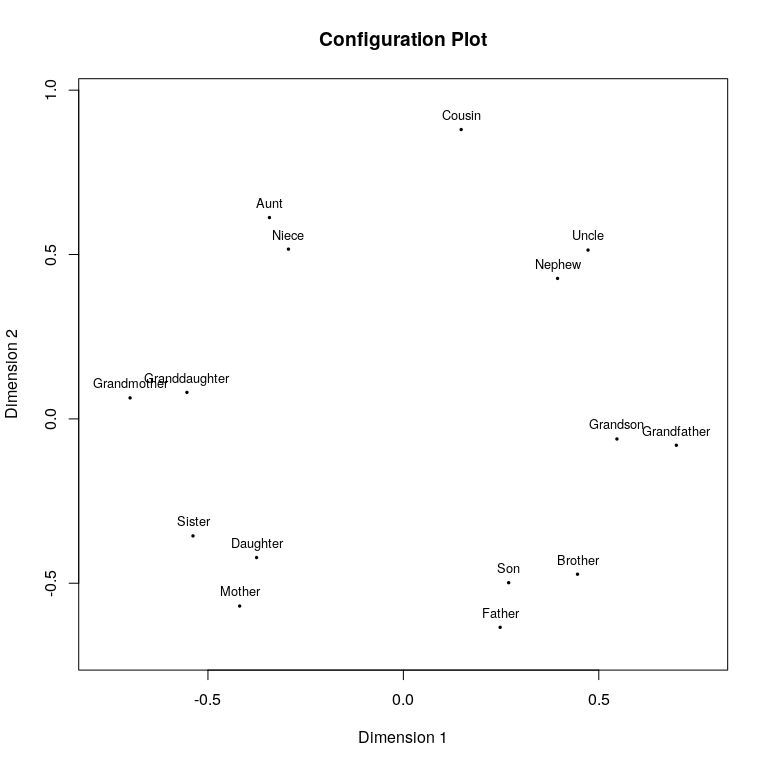We can see that the configuration mimics the structuredness we wanted (there is clusteredness, i.e., the terms are arranged in clusters, the projection is near orthogonal but there is a stochastic dependence that is nonlinear. This is a star-shaped arrangement. (No, this is Patrick). Let us compare this with the corresponding aSTOPS ressa<-stops(kinshipdelta,loss="stress",stressweight=1,structures=c("cclusteredness","clinearity","cdependence"),strucweight=c(-0.33,0.33,-0.33),verbose=0,strucpars=strucpars,type="additive",lower=0,upper=8) ressa ## ## Call: stops(dis = kinshipdelta, loss = "stress", structures = c("cclusteredness", ## "clinearity", "cdependence"), stressweight = 1, strucweight = c(-0.33, ## 0.33, -0.33), strucpars = strucpars, lower = 0, upper = 8, ## verbose = 0, type = "additive") ## ## Model: additive STOPS with stress loss function and theta parameter vector (lambda) = 1.483125 ## ## Number of objects: 15 ## MDS loss value: 0.07037108 ## C-Structuredness Indices: cclusteredness 0.24482385 clinearity 0.02667558 cdependence 0.20393552 ## Structure optimized loss (stoploss): -0.06891657 ## MDS loss weight: 1 c-structuredness weights: -0.33 0.33 -0.33 ## Number of iterations of ALJ optimization: 25 plot(ressa)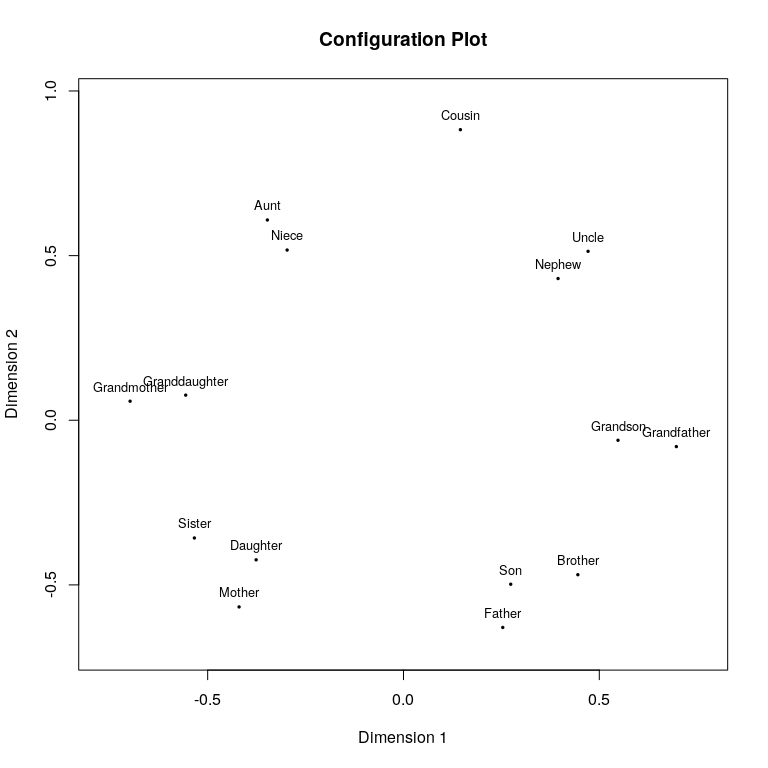Not really different here. When choosing a c-structuredness index, one needs to be clear what structure one might be interested in and how it interacts with the PS loss chosen. Consider the following example: We fit a powermds model to the kinship data and want to maximize c-association (i.e., any non-linear relationship) and c-manifoldness but minimize the c-linearity. In other words we try to find a power transformation of $$\Delta$$ and $$D$$ so that the objects are positioned in the configuration in such a way that the projection onto the principal axes are as close as possible to being related by a smooth but non-linear function. We use tgp as optimization method for itmax=10 steps and restrict the search space to be between $$0$$ and $$5$$. The maximum number of iterations to get the PS configuration is set to itmaxps=1000. set.seed(666) resa<-stops(kinshipdelta,structures=c("cassociation","cmanifoldness","clinearity"),loss="powermds",theta=c(1,1),strucpars=list(NULL,NULL,NULL),type="additive",strucweight=c(-0.5,-0.5,0.5),lower=c(0,0),upper=c(5,5),optimmethod="tgp",itmaxps=1000,itmax=10) resa ## ## Call: stops(dis = kinshipdelta, loss = "powermds", theta = c(1, 1), ## structures = c("cassociation", "cmanifoldness", "clinearity"), ## strucweight = c(-0.5, -0.5, 0.5), strucpars = list(NULL, ## NULL, NULL), optimmethod = "tgp", lower = c(0, 0), upper = c(5, ## 5), type = "additive", itmax = 10, itmaxps = 1000) ## ## Model: additive STOPS with powermds loss function and theta parameter vector (kappa lambda) = 0.4810473 4.443448 ## ## Number of objects: 15 ## MDS loss value: 0.2470057 ## C-Structuredness Indices: cassociation 0.515506235 cmanifoldness 0.956142079 clinearity 0.001926927 ## Structure optimized loss (stoploss): -0.487855 ## MDS loss weight: 1 c-structuredness weights: -0.5 -0.5 0.5 ## Number of iterations of tgp optimization: 10 We see in this model (resa) that indeed the c-manifoldness is almost at 1, which suggests we have the objects arranged close to a manifold structure. c-association is also there and clinearity is near 0, so we see that this is a linear structure. How does this relationship look like? plot(resa)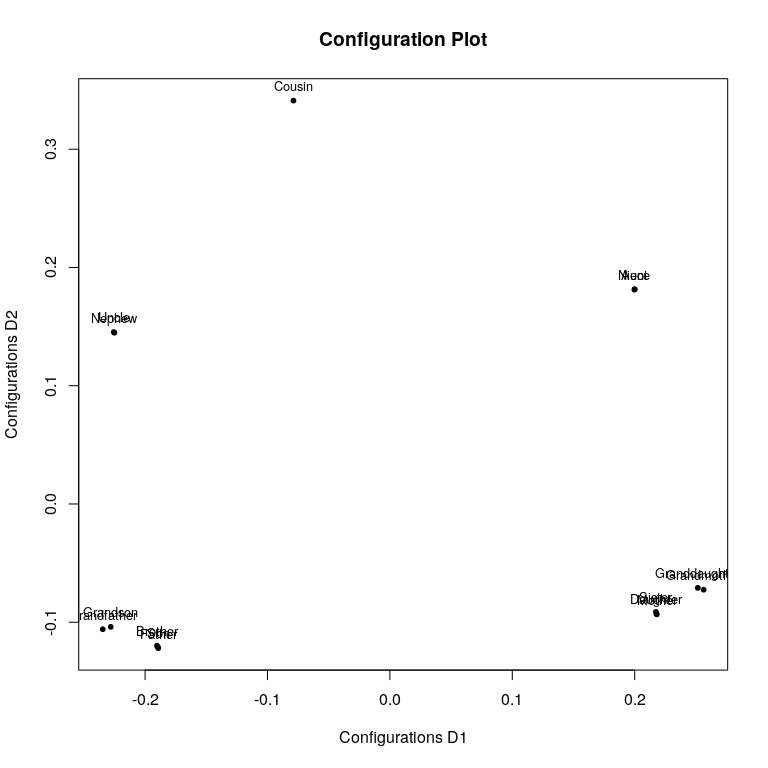The manifold resembles an oval and the objects are arranged in clusters close to that oval. Due to the oval shape the c-association isn’t high as that isn’t really what c-association measures (it measures non-linear curves that do not turn whereas manifoldness ignores bending). What we can also see is that there are three clear clusters of 2 or more objects, but we didn’t select for that. Note that it may just as well be possible to have a high c-association and no c-clusteredness at all (e.g., points lying equidistant on a smooth non-linear curve), as we did not optimize for the latter. The transformation in powermds that is optimal with respect to c-clusteredness could be different. Indeed one can even optimize for c-clusteredness alone (setting stressweight=0) and using it as a “goodness-of-clusteredness” index (i.e., we let the $$d_{max}$$ vary between the configurations and take the highest attainable normed cordillera). This time we use optimmethod="tgp" and itmax=20. set.seed(666) resa2<-stops(kinshipdelta,structures=c("cclusteredness"),loss="powermds",strucpars=list(list(epsilon=10,dmax=NULL,minpts=2)),type="additive",strucweight=-1,stressweight=0,lower=0.1,upper=10,optimmethod="tgp",itmax=20) resa2 ## ## Call: stops(dis = kinshipdelta, loss = "powermds", structures = c("cclusteredness"), ## stressweight = 0, strucweight = -1, strucpars = list(list(epsilon = 10, ## dmax = NULL, minpts = 2)), optimmethod = "tgp", lower = 0.1, ## upper = 10, type = "additive", itmax = 20) ## ## Model: additive STOPS with powermds loss function and theta parameter vector (kappa lambda) = 1.593239 1.593239 ## ## Number of objects: 15 ## MDS loss value: 0.1081298 ## C-Structuredness Indices: cclusteredness 0.7194585 ## Structure optimized loss (stoploss): -0.7194585 ## MDS loss weight: 0 c-structuredness weights: -1 ## Number of iterations of tgp optimization: 20 Then we get a projection with c-clusteredness of 0.7194585. This can of course be done for an c-structuredness. It is also possible to use the stops function for finding the hyperparameters that are optimal for minimizing the badness-of-fit for a given transformation class in the the non-augmented models specified in loss, by setting the strucweight (the weight of any c-structuredness) to 0. Then the function optimizes the MDS loss function only. We use optimmethod="pso" with 5 particles and 100 steps. ressa<-stops(kinshipdelta,theta=1,structures=c("clinearity"),strucweight=0,loss="stress",verbose=0,upper=8,lower=0,optimmethod="pso",itmax=100) Here the minimum badness-of-fit by subjecting the $$\delta$$ to a power transformation $$\delta^*=\delta^\lambda$$ is obtained with $$\lambda^*=$$ and a stress-1 of 0.2652755. This strategy is similar to how the optimal scaling of dhats works within other MDS methods (e.g., in nonmetric and spline MDS). ### COPS A special STOPS model is P-COPS (Rusch, Mair & Hornik 2021). Along the lines of STOPS the overall objective function, which we call , is a weighted combination of the $$\theta-$$parametrized loss function, $$\sigma_{PS}\left(X(\theta)|\theta\right)$$, and a c-clusteredness measure, the OPTICS cordillera or $$OC(X(\theta);\epsilon,k,q)$$ to be optimized as a function of $$\theta$$ or $\begin{equation} \label{eq:spstress} \text{coploss}(\theta) = v_1 \cdot \sigma_{PS}\left(X(\theta) | \theta \right) - v_2 \cdot \text{OC}\left(X(\theta);\epsilon,k,q\right) \end{equation}$ with $$v_1,v_2 \in \mathbb{R}$$ controlling how much weight should be given to the scaling fit measure and the c-clusteredness. The c-clusteredness index we use is the OPTICS cordillera which measures how clustered a configuration appears. It is based on the OPTICS algorithm that outputs an ordering together with a distance. The OPTICS cordillera is now simply an agregation of that information. Since we know what constitutes a maximally clustered result, we can derive an upper bound and normalize the index to lie between 0 and 1. If it is maximally clustered, the index gets a value of 1,and it gets 0 if all points are equidistant to their nearest neighbours (a matchstick embedding). See the vignette in the COPS package for more. ### A Full Blown Analysis We recreate parts of an example of Rusch, Mair and Hornik (2023) here. The data are 500 handwritten pen digits and we subject them to different flexible MDS methods and select hyperparameters with STOPS based on c-clusteredness and c-manifoldness. We use Sammon mapping with power transformations for the proximities, Box-Cox MDS, lMDS (all three like in the article) and also approximate power stress (different from the article). The data are available in the package via data(Pendigits500). This is a tough data set for our implementations because it is quite large, so an individual MDS takes a while. When running STOPS we have to fit a large number of MDS, so this can take quite a long time and therefore we also time so that users can see what to expect. # data setup data(Pendigits500) pendss <- Pendigits500 names(pendss) <- "digit" ## setup data and the c-structuredness hyperparameters dis <- dist(pendss[,1:16]) #only features are used to get the dissimilarity matrix, not the digit label # parameters for OC (c-clusteredness) q <- 2 rang <- c(0,0.6) minpts <- 5 eps <- 10 scale <- 3 We start with an initial solution (a Sammon mapping) and see how the c-structuredness of that result is ## initial sammon mapping solution initsam <- sammon(dis) ## Initial stress : 0.15233 ## stress after 1 iters: 0.15079 ## c-structuredness of initial solution c1 <- cordillera::cordillera(initsam$points,minpts=minpts,q=q,epsilon=eps,rang=rang,scale=scale)
ccom1 <- c_manifoldness(initsam$points) c1 ## raw normed ## 0.70519137 0.08331615 ccom1 ##  0.593913 So the c-clusteredness was 0.0833162 and c-manifoldness was 0.593913. This is our reference solution to see if we can improve c-structuredness. Next we set up the structures and the parameters for the structuredness indices # #c-structuredness metaparameters strucpars <- list(list(minpts=minpts,epsilon=eps,rang=rang,scale=scale), #cordillera list(alpha=0.9,C=15,var.thr=1e-5,zeta=NULL) #manifoldness ) structures <- c("cclusteredness","cmanifoldness") #structures We use weights of $$-400$$ for c-clusteredness and $$-2.5$$ for c-manifoldness. ## c-structuredness weights strucweight <- c(-400,-2.5) Now we have everything to run STOPS. We use Sammon mapping with powers for the dissimilarities, local MDS and Box-Cox MDS. The search space is from $$1$$ to $$6$$ and we use optimmethod='tgp' (one can set verbose=4 to see details of the iteration progress). We also time the calculations. #Sammon with power transformations of proximities set.seed(666) timesam <- system.time(restgp <- stops(dis,theta=5.6,loss="sammon",structures=structures,stressweight=1,strucweight=strucweight,strucpars=strucpars,lower=1,upper=6,optimmethod="tgp",model="btgpllm",itmax=10)) #Box Cox MDS set.seed(667) timebc <- system.time(restgpbc <- stops(dis,theta=c(4.63,2.77,5.12),loss="bcstress",structures=structures,stressweight=1,strucweight=strucweight,strucpars=strucpars,lower=c(0.1,0.1,0.1),upper=c(6,6,6),optimmethod="tgp",model="btgpllm",itmax=10,itmaxps=1e4)) #lMDS set.seed(669) timelmds <- system.time(restgplmds <- stops(dis,theta=c(14.5,3),loss="lmds",weightmat=dis,structures=structures,stressweight=1,strucweight=strucweight,strucpars=strucpars,lower=c(5,0),upper=c(50,3),optimmethod="tgp",model="btgpllm",itmax=10,itmaxps=1e4)) #approx p stress set.seed(670) timeaps <- system.time(restgpaps <- stops(dis,theta=c(0.53,2.85),loss="apstress",structures=structures,stressweight=1,strucweight=strucweight,strucpars=strucpars,lower=c(0.1,0.1),upper=c(6,6),optimmethod="ALJ",itmax=20,itmaxps=1e4)) We now plot the results. We color the digits differently and adjust all the configurations to the same plotting region via Procrustes transformation (as MDS results are invariant to translation, scaling and rotation). #plot the digit results colsopt <- cols1 <- colstraj <- factor(pendss[,17]) levels(colsopt) <- hcl.colors(10,"Dark 3") #Procrustes adjustment to lmds configuration for STOPS optimized samconf <- restgp$fit$conf lmdsconf <- restgplmds$fit$conf bcconf <- restgpbc$fit$conf apsconf<-restgpaps$fit$conf samconf <- Procrustes(lmdsconf,samconf)$Yhat
bcconf <- Procrustes(lmdsconf,bcconf)$Yhat apsconf <- Procrustes(lmdsconf,apsconf)$Yhat

And here are the plots.

par(mfrow=c(2,2))
#Sammon
plot(samconf[,1],samconf[,2],col=as.character(colsopt),type="n",xlab="",ylab="",asp=1,axes=TRUE,main="Sammon")
points(samconf[,1],samconf[,2],col=as.character(colsopt),pch=as.character(pendss[,17]))
box()

#lmds
plot(lmdsconf[,1],lmdsconf[,2],col=as.character(colsopt),type="n",xlab="",ylab="",asp=1,main="lMDS",axes=TRUE)
points(lmdsconf[,1],lmdsconf[,2],col=as.character(colsopt),pch=as.character(pendss[,17]))
box()

#bc stress
plot(bcconf[,1],bcconf[,2],col=as.character(colsopt),type="n",xlab="",ylab="",asp=1,axes=TRUE,main="BC-MDS")
points(bcconf[,1],bcconf[,2],col=as.character(colsopt),pch=as.character(pendss[,17]))
box()

#ap stress
plot(apsconf[,1],apsconf[,2],col=as.character(colsopt),type="n",xlab="",ylab="",asp=1,axes=TRUE,main="Approx. POST-MDS")
points(apsconf[,1],apsconf[,2],col=as.character(colsopt),pch=as.character(pendss[,17]))
box()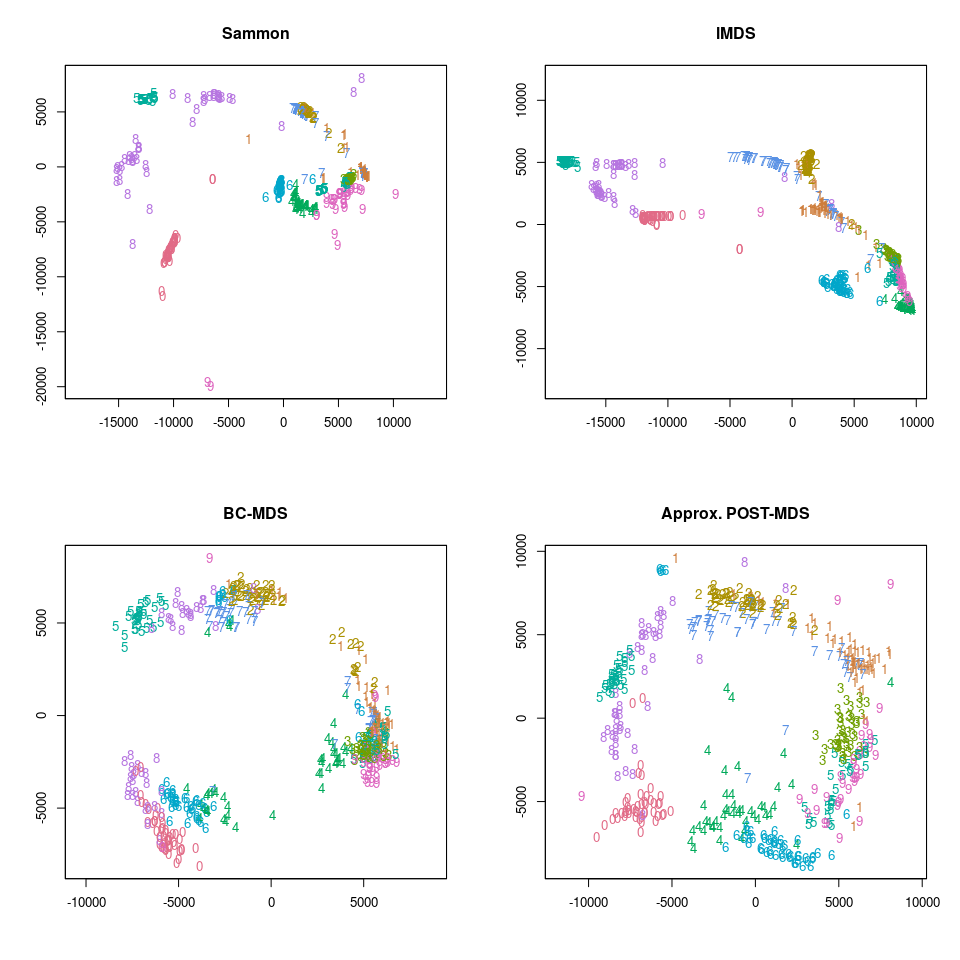We can see that Sammon mapping with power transformation shows a clusteredness that is both high and sensible. The values is 0.1500707 for Sammon. Box-Cox MDS also give s arelatively clustered result, but it seems it finds 3 clusters and that is less in line wht the ground truth. The value is 0.1240366.

restgp
##
## Call: stops(dis = dis, loss = "sammon", theta = 5.6, structures = structures,
##     stressweight = 1, strucweight = strucweight, strucpars = strucpars,
##     optimmethod = "tgp", lower = 1, upper = 6, verbose = 4, itmax = 10,
##     model = "btgpllm")
##
## Model: additive STOPS with sammon loss function and theta parameter vector (lambda)  =  5.399645
##
## Number of objects: 500
## MDS loss value: 0.6970387
## C-Structuredness Indices: cclusteredness 0.1500707 cmanifoldness 0.9280689
## Structure optimized loss (stoploss): -61.6514
## MDS loss weight: 1 c-structuredness weights: -400 -2.5
## Number of iterations of tgp optimization: 10
restgpbc
##
## Call: stops(dis = dis, loss = "bcstress", theta = c(4.63, 2.77, 5.12),
##     structures = structures, stressweight = 1, strucweight = strucweight,
##     strucpars = strucpars, optimmethod = "tgp", lower = c(0.1,
##         0.1, 0.1), upper = c(6, 6, 6), verbose = 4, itmax = 10,
##     itmaxps = 10000, model = "btgpllm")
##
## Model: additive STOPS with bcstress loss function and theta parameter vector (mu lambda rho)  =  4.631187 4.85914 1.285368
##
## Number of objects: 500
## MDS loss value: 0.1293095
## C-Structuredness Indices: cclusteredness 0.1240366 cmanifoldness 0.9610854
## Structure optimized loss (stoploss): -51.88803
## MDS loss weight: 1 c-structuredness weights: -400 -2.5
## Number of iterations of tgp optimization: 10

Local MDS and approximate power stress give configurations that are less clustered, although the arrangement of points is still sensible even if they don’t align in clusters.

restgpbc
##
## Call: stops(dis = dis, loss = "bcstress", theta = c(4.63, 2.77, 5.12),
##     structures = structures, stressweight = 1, strucweight = strucweight,
##     strucpars = strucpars, optimmethod = "tgp", lower = c(0.1,
##         0.1, 0.1), upper = c(6, 6, 6), verbose = 4, itmax = 10,
##     itmaxps = 10000, model = "btgpllm")
##
## Model: additive STOPS with bcstress loss function and theta parameter vector (mu lambda rho)  =  4.631187 4.85914 1.285368
##
## Number of objects: 500
## MDS loss value: 0.1293095
## C-Structuredness Indices: cclusteredness 0.1240366 cmanifoldness 0.9610854
## Structure optimized loss (stoploss): -51.88803
## MDS loss weight: 1 c-structuredness weights: -400 -2.5
## Number of iterations of tgp optimization: 10
restgpaps
##
## Call: stops(dis = dis, loss = "apstress", theta = c(0.53, 2.85), structures = structures,
##     stressweight = 1, strucweight = strucweight, strucpars = strucpars,
##     optimmethod = "ALJ", lower = c(0.1, 0.1), upper = c(6, 6),
##     verbose = 4, itmax = 20, itmaxps = 10000)
##
## Model: additive STOPS with apstress loss function and theta parameter vector (tau upsilon)  =  0.5091412 2.842567
##
## Number of objects: 500
## MDS loss value: 0.05392559
## C-Structuredness Indices: cclusteredness 0.08691348 cmanifoldness 0.81720840
## Structure optimized loss (stoploss): -36.75449
## MDS loss weight: 1 c-structuredness weights: -400 -2.5
## Number of iterations of ALJ optimization: 20

This brings us to c-manifoldness which is very high for Sammon, Box-Cox MDS and lMDS. Approximate power stress MDS (POST-MDS) shows c-manifoldness that is a bit less bit still relatively high (it is less mainly due ot being more noisy around the imagined manifold). The manifolds are alos easy to see: for Sammon it seems to be reminiscent of a spiral, for approximate power stress it is a circle, for Box-Cox MDS it is a circle as well (or three spherical clusters) and for lMDS a manifold is also apparent although I don’t think it has a name.

The interesting thing here is that the objects are arrnaged along the manifolds in a way that suggests a structure of how handwritten digits are related, e.g., that 0s and 8s are related and that they are closer to 5s than to 1s. There is also a way to get from one digit to others along the manifold, e.g, in approx. POST-MDS one would get from the 6s to over the 9s to the 3s. This may give a hypothesis about a relationship amongst them.

### Extras

We also briefly describe some other functions in the package stops.

Since the inner optimization problem in STOPS models is hard and takes long, Rusch, Mair and Hornik (2021) developed a metaheuristic for the outer optimization problem that typically needs less calls to the inner minimization than SANN, albeit without the guarantees of convergence to a global minimum for non-smooth functions. It is an adaptation of the Luus-Jaakola random search (Luus & Jaakola 1973). It can used with the function ljoptim which modeled its output after optim. It needs as arguments x a starting value, fun a function to optimize, a lower and upper box constraint for the search region. By using the argument adaptive=TRUE or FALSE one can switch between our adaptive version and the original LJ algorithm. Accuracy of the optimization can be controlled with the maxit (maximum number of iterations), accd (terminates after the length of the search space is below this number ) and acc arguments (terminates if difference of two subsequent function values are below this value).

We optimize a “Wild Function” with the non-adaptive LJ version (and numerical accuracies of at least 1e-16 for accd and acc).

set.seed(210485)
fwild <- function (x) 10*sin(0.3*x)*sin(1.3*x^2) + 0.00001*x^4 + 0.2*x+80
res2
## $par ##  -15.81515 ## ##$value
##  67.46773
##
## $counts ## function gradient ## 463 NA ## ##$convergence
##  0
##
## $message ## NULL plot(fwild, -50, 50, n = 1000, main = "ljoptim() minimising 'wild function'") points(res2$par,res2\$value,col="red",pch=19)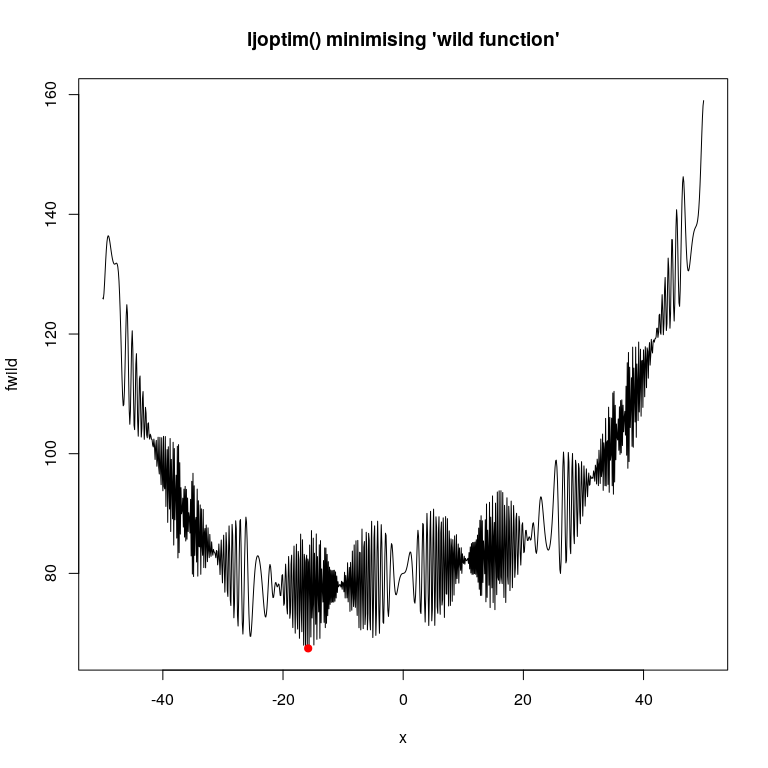We also provide a procrustes adjustment to make two configurations visually comparable. The function is conf_adjust and takes two configurations conf1 the reference configuration and conf2 another configuration. It returns the adjusted versions
conf_adjust(conf1,conf2)